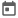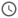# Queues in system verilog##### 12 Mar 2022##### 4 mins

Queue is a special data type in System Verilog which works on the principle of FIFO (First in First Out). Thus, the element which is stored first in the queue is retrieved first, just as the case in real-life queue. Let’s see queue in depth on this article.

### What is Queue?

Queue are based on the concept of linked list in which the elements consist of 2 parts. 1st part is the value that needs to be stored, and the 2nd part is the address of the next element of the list. If the element is last one, then the 2nd part is null otherwise it points to the next item.

Queue in system Verilog, handles all the address related operations internally which makes using queue a lot easier. Queue like an associative array has no specific size and the size grows and shrinks dynamically depending upon the number of elements stored. But in queues the index is continuous in nature unlike the associative arrays.

#### Concept of push and pop in queue

As discussed, queues work on the concept of FIFO, i.e., first in first out. This means that a new element can be added at the end of the queue. Also, the element which is first in queue means that it was inserted earlier than other elements and thus this element will be removed from the queue first.

• Push in queue means inserting a new element at the end of the queue.
• Pop in queue means getting the first element present in the queue and removing it from the queue.

#### Syntax

Declaration of queue is like that of the dynamic or associative array, but `\$` is used inside the square brackets. `\$` symbol differentiates a queue from dynamic or associative array. Also, `\$` symbol indicates the last element and can be used to refer to the last element of the array

``````<element datatype> <queue_name> [\$];

// Example
int first_queue [\$];``````

#### Example

``````module queue_example();
bit[7:0] queue [\$]; // queue which can hold 8-bit data
initial begin
queue[\$+1] = 8'd21;
queue[\$+1] = 8'd45;
queue[\$+1] = 8'd87;
queue[\$+1] = 8'd159;
queue[\$+1] = 8'd77;
\$display("Values stored in queue are %p", queue);

queue = '{8'd45, 8'd77, 8'd159, 8'd12};
\$display("Values stored in queue are %p", queue);
end
endmodule``````
##### Output
``````# Values stored in queue are '{ 21, 45, 87, 159, 77 }
# Values stored in queue are '{ 45, 77, 159, 12 }``````
Try this code in EDA Playground
Using `\$` symbol for adding new elements in the queue may not produce the same result in different simulator. Thus, it is recommended to use the in-built functions to add or remove elements from a queue so that the output is same on all simulators.

### In-built functions for Queues

System Verilog provides various in-build functions which make working with queues a lot easier. Also, it encapsulates lot of complex operation which is involved in queue operations.

1. `int size()` - returns the number of elements present in the queue.
2. `element_t pop_front()` - returns the first element present in the queue and deletes it.
3. `element_t pop_back()` - returns the last element present in the queue and deletes it.
4. `void push_front(element_t item)` - Inserts the item in the first position of the queue. Index of all other item shifts by 1.
5. `void push_back()` - inserts the item in the last position of the queue. All other items present in the queue are unaffected.
6. `void insert(int index, element_t item)` - inserts the item in the specified index.
7. `void delete([int index])` - if index is provided as input, it deletes the item present in specific index otherwise it delete the entire queue.

#### Example

``````module queue_func_example();
bit [7:0] queue [\$]; // queue which can hold 8-bit data

initial begin
\$display("Number of elements present in queue in %0d", queue.size());
queue.push_back(34);
queue.push_back(67);
queue.push_back(123);
\$display("Values stored in queue after push_back are %p", queue);

queue.push_front(212);
\$display("Values stored in queue after push_front are %p", queue);

\$display("Number of elements present in queue in %0d", queue.size());

\$display("Poped value from back = %0d", queue.pop_back());
\$display("Values stored in queue after pop_back are %p", queue);

\$display("Poped value from front = %0d", queue.pop_front());
\$display("Values stored in queue after pop_back are %p", queue);

// Note that index starts from 0
queue.insert(1, 56);
\$display("Values stored in queue after insert at 2nd position are %p", queue);

queue.delete(1);   // deletes item at index 1
\$display("Values stored in queue after deleting item at 1st position are %p", queue);

queue.delete();    // delets all the item
\$display("Values stored in queue after deleting all item are %p", queue);
end
endmodule``````
##### Output
``````# Number of elements present in queue in 0
# Values stored in queue after push_back are '{34, 67, 123}
# Values stored in queue after push_front are '{212, 34, 67, 123}
# Number of elements present in queue in 4
# Poped value from back = 123
# Values stored in queue after pop_back are '{212, 34, 67}
# Poped value from front = 212
# Values stored in queue after pop_back are '{34, 67}
# Values stored in queue after insert at 2nd position are '{34, 56, 67}
# Values stored in queue after deleting item at 1st position are '{34, 67}
# Values stored in queue after deleting all item are '{}``````
Try this code in EDA Playground

### Example use case

Let’s suppose there is a process which samples data and send it to the processing unit. It is not necessary that the data sent by the sampling unit would be processed immediately.

In this scenario we don’t know exactly how many elements we need to store thus dynamic array is not a good option. Also, associative array is not good for this scenario as we don’t need the elements after the element has been processed. In case of associative array, the size will increase, and it is slower.

Thus, queues are the best option for this scenario as sampling unit can push data once it has sampled it and processing unit can pop it whenever it can process the data.

``````module queue_example2();
bit [7:0] buffer [\$]; // queue which can hold 8-bit data

// Sampling unit
always begin
sampled_data = \$urandom_range(255);
\$display("[%0t]Sampled value is %0d\n", \$time, sampled_data);
buffer.push_back(sampled_data);
#10;
end

// processing unit
always begin
\$display("[%0t]Number of elements present in queue in %0d", \$time, buffer.size());
\$display("[%0t]Values stored in buffer is %p", \$time, buffer);
\$display("[%0t]Recieved value is %0d\n", \$time, received_data);
#20;
end
endmodule``````
##### Output
``````# Sampled value is 184
#
# Number of elements present in queue in 1
# Values stored in buffer is '{184}
# Recieved value is 184
#
# Sampled value is 38
#
# Number of elements present in queue in 1
# Values stored in buffer is '{38}
# Recieved value is 38
#
# Sampled value is 102
#
# Sampled value is 182
#
# Number of elements present in queue in 2
# Values stored in buffer is '{102, 182}
# Recieved value is 102
#
# Sampled value is 198
#
# Sampled value is 70
#
# Number of elements present in queue in 3
# Values stored in buffer is '{182, 198, 70}
# Recieved value is 182
#
# Sampled value is 114
#
# Sampled value is 46
#
# Number of elements present in queue in 4
# Values stored in buffer is '{198, 70, 114, 46}
# Recieved value is 198
#
# Sampled value is 103``````
Try this code in EDA Playground